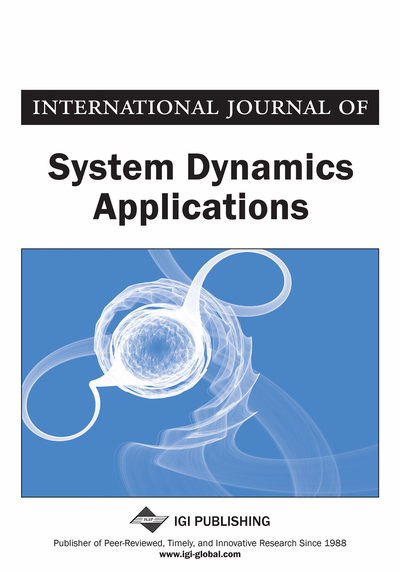# An Analysis of a Wind Turbine-Generator System in the Presence of Stochasticity and Fokker-Planck Equations

Ravish Himmatlal Hirpara (S.V. National Institute of Technology (SVNIT), Surat, India) and Shambhu Nath Sharma (S.V. National Institute of Technology (SVNIT), Surat, India)
DOI: 10.4018/IJSDA.2020010102
Available
\$37.50
No Current Special Offers

## Abstract

In power systems dynamics and control literature, theoretical and practical aspects of the wind turbine-generator system have received considerable attentions. The evolution equation of the induction machine encompasses a system of three first-order differential equations coupled with two algebraic equations. After accounting for stochasticity in the wind speed, the wind turbine-generator system becomes a stochastic system. That is described by the standard and formal Itô stochastic differential equation. Note that the Itô process is a strong Markov process. The Itô stochasticity of the wind speed is attributed to the Markov modeling of atmospheric turbulence. The article utilizes the Fokker-Planck method, a mathematical stochastic method, to analyse the noise-influenced wind turbine-generator system by doing the following: (i) the authors develop the Fokker-Planck model for the stochastic power system problem considered here; (ii) the Fokker-Planck operator coupled with the Kolmogorov backward operator are exploited to accomplish the noise analysis from the estimation-theoretic viewpoint.
Article Preview
Top

## 1. Introduction

The wind energy has become one of the popular renewable sources for the generation of the electrical power. Three different configurations are popular in the wind power conversion technology, the fixed speed wind turbine-generator system, the limited variable speed wind turbine-generator system and variable speed wind turbine-generator system. The main components of the fixed speed wind turbine-generator system are wind turbine, gearboxes, squirrel cage induction generator, capacitor. That are connected to the grid through the transformer. This configuration allows the machine to run at the constant speed as well as provides stable frequency. Secondly, the limited variable speed wind turbine-generator system utilizes the wound rotor induction generator in place of the squirrel cage. This structure utilizes a power electronic converter. Thirdly, the variable speed wind turbine-generator system accounts for the doubly-fed induction generator with a power electronic converter (Li and Chen, 2008). Here, some important dynamical equations for the wind turbine-generator system are described. Fu and Xing (2009) have developed a four-dimensional Squirrel Cage Induction Generator (SCIG) model. Subsequently, a reduced-order model was developed using singular perturbation method. The singular perturbation method hinges on the electrical engineering assumption that stator transients are faster than rotor transients. Thus, the evolutions of stator transients are not accounted for and the evolutions of rotor transients are accounted for. Taha Hussien et al. (2017) have developed modeling of three phase induction motor and explain stator turns fault analysis based on artificial intelligence. Salima et al. (2018) have developed a Global stability of linearizing control of induction motor for PV water pumping application. To study the control system design using Matlab see (Moysis et al. 2017). Gabhi et al. (2018) have developed discrete sliding mode control scheme for nonlinear systems with bounded uncertainties and Alain et al. (2017) have developed improved robust adaptive control strategy for the finite time synchronization of uncertain nonlinear systems. Feijóo et al. (2000) have considered a third-order deterministic equation that explains modelling of the rotor transient voltages behind the transient impedance. Balanathan et al. (2002) have developed a dynamic load model. That has ability to analyse the transient and steady state stabilities of the induction motor. To study the influence of the wind power dynamics on the large electrical power systems dynamics and control, Slootweg et al. (2003) argue the addition of wind turbine-generating systems’ models in software packages, especially for numerical experimentations. In this connection, especially for numerical experimentations of wind turbine models, two articles, i.e. Sorensen et al. (2002) and Nichita et al. (2002), would be also useful. Dusonchet et al. (2007) have investigated the influence of wind turbine mechanical characteristics. The transient voltage stability of the fixed speed wind turbine-generator systems were studied as well. In their investigation, the fifth-and the third-order models were the subject of investigations. Generally, the third-order model is a special case of the fifth-order model. The third-order does not account for stator transients into considerations, see Popović et al. (1998) and Ledesma et al. (2003). Furthermore, Martins et al. (2007) have validated the fifth-and third-order models with measured data, which are rarely reported in literature. The preceding introductory descriptions of this paper suggest that the problem of analysing the wind turbine-generator system from the dynamical systems’ viewpoint received considerable attention in literature. Under the wind turbulence influence, it is reasonable to account for the stochastic character of the wind speed for the wind turbine-generator system in lieu of the deterministic formalism. A good discussion about the wind turbine-generator system under stochastic influence can be found in brief research writing, but a compelling paper in stochastic sense (Wang and Crow 2012). The stochastically perturbed dynamical system involves investigations into vector Stochastic Differential Equations (SDEs) in lieu of Ordinary Differential Equations (ODEs). This seems to be one of the reasons that the notion of stochastic processes is relatively very less introduced into ‘power systems dynamics’ literature yet.

## Complete Article List

Search this Journal:
Reset
Volume 10: 4 Issues (2021): 2 Released, 2 Forthcoming
Volume 9: 4 Issues (2020)
Volume 8: 4 Issues (2019)
Volume 7: 4 Issues (2018)
Volume 6: 4 Issues (2017)
Volume 5: 4 Issues (2016)
Volume 4: 4 Issues (2015)
Volume 3: 4 Issues (2014)
Volume 2: 4 Issues (2013)
Volume 1: 4 Issues (2012)
View Complete Journal Contents Listing